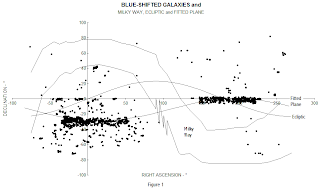## Thursday, December 10, 2009

BLUE SHIFTED GALAXIES
There are more almost 7000 blue-shifted galaxies listed in the NED1 web site. Being blue-shifted means that they are approaching the earth. The velocities ranges from just-above-zero to 8000 km/sec. The vast majority of velocities is less than 200 km/sec.

In Figure 1, the location of a random sample (2000) of these galaxies is shown in relation to the Milky Way (the Galaxy) and the ecliptic About 90% of the blue-shifted galaxies are located in two groups which are located approximately 180º apart.

In Figure 1, you can see that the image of the Milky Way2 passes between the two groupsof blue-shifted galaxies. The plane of the Galaxy is at a steep angle of about 60º. In other words, the solar system disk “sticks out” of the Galactic plane in two places around RA 0º (to the south) and RA 180º (to the north). Otherwise, it is pretty much shrouded by the Milky Way.

Its hard to see distant galaxies through the Milky Way disk because its light masks them. Open space is accessible to the solar system where it is trying to “stick out”. The other regions experience significant interference from the Milky Way.

In Figure 2 a sample (smaller) of blue-shifted galaxies is plotted on the celestial sphere as seen from the outside. You can see that the dense band of galaxies is located on a plane, B, that is fitted to the edge of the band of blue-shifted galaxies. The normal to the plane is marked A in Figure 2.Figure 2
Showing the blue-shifted galaxies (dots), the plane fitted to the principal band (B), and the normal to the band (A).. Right Ascensions are marked on the celestial equator. The point from which the celestial sphere is viewed: (∞, RA 359.83°, Decl 4.62°).

The parameters (A = 1, RA 15.8°, Decl 75.5°; b = -0.234) for a best-fit plane for the band of blue-shifted galaxies were calculated using the equation:

x·A = b

where: x is the unit vector to the position of the galaxy,
A is the unit vector perpendicular to the plane,
b is the displacement of the plane from the celestial origin.

The fitted plane can be thought of as being a plane that intersects the celestial sphere at Decl -13.5° (the arcsine of -0.234) which is, then, tipped from the z-axis by 14.5° and rotated around it by 15.8°.

Some other bands (with much smaller number of galaxies) seem to be suggested, also. One coincides with the celestial equator.

CONCLUSION
In the last two year almost 200 blue-shifted galaxies have been added to the NED web site—most likely credit to the Hubble telescope. The pattern that they form in the sky seems to be a useful tool to explore our universe.

1 This research has made use of the NASA/IPAC Extragalactic Database (NED) which is operated by the Jet Propulsion Laboratory, California Institute of Technology, under contract with the National Aeronautics and Space Administration.
http://nedwww.ipac.caltech.edu/cgi-bin/nph-allsky?ra_constraint=Unconstrained&ra_1=&ra_2=&dec_constraint=Unconstrained&dec_1=&dec_2=&glon_constraint=Unconstrained&glon_1=&glon_2=&glat_constraint=Unconstrained&glat_1=&glat_2=&z_constraint=Less+Than&z_value1=0&z_value2=&z_unit=km%2Fs&ot_include=ANY&ex_objtypes1=Clusters&ex_objtypes1=Supernovae&ex_objtypes1=QSO&ex_objtypes2=AbsLineSys&ex_objtypes2=GravLens&ex_objtypes2=Radio&ex_objtypes2=Infrared&ex_objtypes3=EmissnLine&ex_objtypes3=UVExcess&ex_objtypes3=Xray&ex_objtypes3=GammaRay&nmp_op=ANY&out_csys=Equatorial&out_equinox=B1950.0&obj_sort=RA+or+Longitude&zv_breaker=30000.0
2 Star Map in Galactic Perspective http://www.datasync.com/~rsf1/fun/sm-new.htm